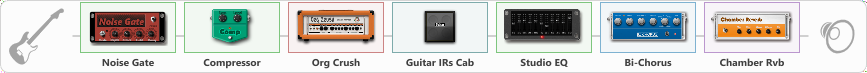# Orange ZZap

Discussion in 'ToneLib-GFX presets' started by Seattlesauce, Mar 7, 2021.

1. Orange ZZap

Preset name: Orange Zzzap

Effects chain:Effect: "Noise Gate" (Dynamics / Filter), active - "yes"
{
"Mode" = Auto
"Depth" = 65
"Threshold" = 71
"Attack" = 8
"Hold" = 495
"Decay" = 250
}

Effect: "Compressor" (Dynamics / Filter), active - "yes"
{
"Sense" = 78
"Level" = 76
}

Effect: "Org Crush" (Amp simulators), active - "yes"
{
"Gain" = 70
"Bass" = 51
"Middle" = 40
"Treble" = 46
"Presence" = 44
"Master" = 75
"Level (dB)" = 0
}

Effect: "Guitar IRs Cab" (Cabinets), active - "yes"
{
"Model" = Orange PPC (4x12")
"Mic Position" = Middle
"Mic Distance" = Middle
"Low Cut (Hz)" = 78
"Hi Cut (kHz)" = 17.5
"Mix" = 100
"Level (dB)" = 0
}

Effect: "Studio EQ" (Dynamics / Filter), active - "yes"
{
"31 Hz" = -5
"62 Hz" = 2
"125 Hz" = 0
"250 Hz" = 0
"500 Hz" = 1
"1 kHz" = 1
"2 kHz" = -1
"4 kHz" = 1
"8 kHz" = 0
"16 kHz" = -5
"Level (dB)" = 2
}

Effect: "Bi-Chorus" (Modulation / Sfx), active - "yes"
{
"Speed A" = 3.4
"Speed B" = 2.8
"Depth" = 24
"Reso" = 3
"Mode" = Parallel
"Mix" = 68
}

Effect: "Chamber Rvb" (Reverberation), active - "yes"
{
"Time" = 3.1
"PreDelay" = 30
"LoDamp" = 41
"HiDamp" = 46
"Mix" = 58
}

Note: You will need to download and install the ToneLib-GFX software to use the preset.

File size:
757 bytes
Views:
2,470